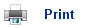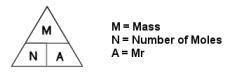Chemical FormulaeTo work out the number of moles in an element/compound, just divide the mass by the Ar or the Mr.

For example:

“How many moles are there in 32g of oxygen?”

Ar of oxygen is 16

So 32/16 = number of moles

Number of moles = 2

To work out the mass, multiply the number of moles by the Ar or Mr.

For example:

“What is the mass of 6 moles of Carbon?”

Ar of Carbon is 12

So 12 x 6 = Mass

Mass= 72g

• Avagadro's constant = the number of atoms is a mole of an element = 6.02 x 1023
• To work out the number of particles, just multiply the number of moles by Avagadro's constant

For example:

“How many particles are there in 2 moles of carbon?”

2 x 6.02 x1023

= 1.2 x 1024

• Empirical Formula- the simplest ratio of atoms of each element in a compound.
• Molecular Formula- the actual number of each atom in a molecule.
• To work out the empirical formulae of a substance, the mass is needed.

For example:

“0.020g of Hydrogen combine with 0.168g of Oxygen. What is the empirical formula of Water?”

Mass:  0.020g H                    0.168g O

Number of Moles: 0.20/1    0.168/16

Ratio: 0.02 : 0.01 = 2:1 so the formula is H2O

To work out the molecular formula, divide the Molecular Mass by the sum of the Atomic masses of the empirical formula.

For example:

“The empirical formula of methane is CH3, the formula mass of methane is 30, work out the molecular formula”

CH3 = 12 + (3x1)

= 15

30/15= 2

so the molecular formula is twice the empirical formula.

C2H6

## Useful books for revision:

Revise AS Chemistry for Salters (Written by experienced examiners and teachers of Salter's chemistry)Revise AS Chemistry for Salters (OCR) (Salters Advanced Chemistry)Home